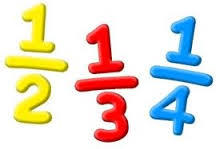Simplest Form
4 years ago
lauracarrier
Save
Edit
Host a game
Live GameLive
Homework
Solo Practice
Practice10 QuestionsShow answers
• Question 1
30 seconds
Q. A fraction is in simplest form when the numerator and denominator
are the same
are both even
are both odd
have no common factor other than 1
• Question 2
30 seconds
Q. To put a fraction in simplest form, you will need to do this to the numerator and denominator
subtract both by same number
divide both by the same number
add the same number to both
multiply both by the same number
• Question 3
30 seconds
Q. Put 6/8 in simplest form.
3/4
12/16
1/4
60/80
• Question 4
30 seconds
Q. All even numbers have _____ as a factor.
3
5
2
4
• Question 5
30 seconds
Q. Put 3/5 in simplest form.
1/3
6/10
2/3
• Question 6
30 seconds
Q. Put 3/12 in simplest form.
6/24
1/4
1/3
• Question 7
30 seconds
Q. Put 6/10 in simplest form.
3/6
12/20
3/5
• Question 8
30 seconds
Q. Put 10/100 in simplest form.
1/10
5/50
2/20
• Question 9
30 seconds
Q. Putting a fraction in simplest form is sometimes referred to as
reducing the fraction
multiplying the fraction